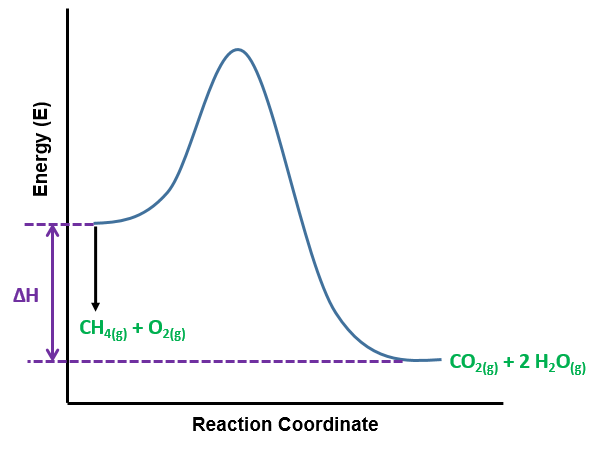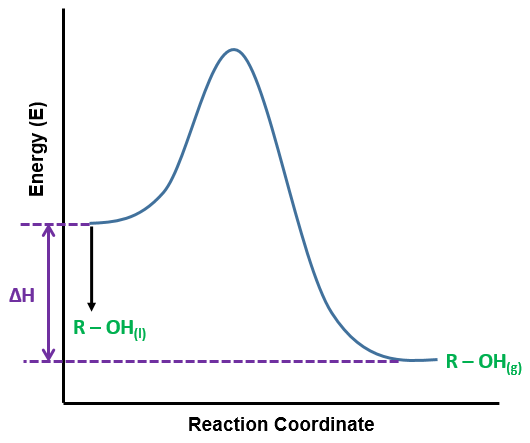# Problem: Write a balanced equation and draw an enthalpy diagram for (select if exothermic or endothermic):combustion of one mole of methanevaporization of liquid alcoholfreezing of liquid waterformation of 1 mole of potassium chloride from its elements (heat is released)

###### FREE Expert Solution

Exothermic reactions

▪ lose energy, releases heat, temperature of the surroundings increases
▪ involves bond formation (for phase change: gas → liquid → solid)
▪ ΔH is negative

Endothermic reactions

▪ gain/requires energy, absorbs heat, temperature of the surroundings decreases
▪ involves breaking bonds (for phase change: solid → liquid → gas)
▪ ΔH is positive

• combustion of one mole of methane

Reaction:      CH4(g) + 2 O2(g) → CO2(g) + 2 H2O(g)

▪ releases heat:   exothermic• vaporization of liquid alcohol

*generic form of alcohol: R‒OH

Reaction:      R‒OH(l) → R‒OH(g)

▪ phase change (liquid → gas):    endothermic• freezing of liquid water

Reaction:      H2O(l) → H2O(s)

87% (416 ratings)###### Problem Details

Write a balanced equation and draw an enthalpy diagram for (select if exothermic or endothermic):

combustion of one mole of methane

vaporization of liquid alcohol

freezing of liquid water

formation of 1 mole of potassium chloride from its elements (heat is released)

Frequently Asked Questions

What scientific concept do you need to know in order to solve this problem?

Our tutors have indicated that to solve this problem you will need to apply the Energy Diagram concept. If you need more Energy Diagram practice, you can also practice Energy Diagram practice problems.

What professor is this problem relevant for?

Based on our data, we think this problem is relevant for Professor Harris' class at UF.